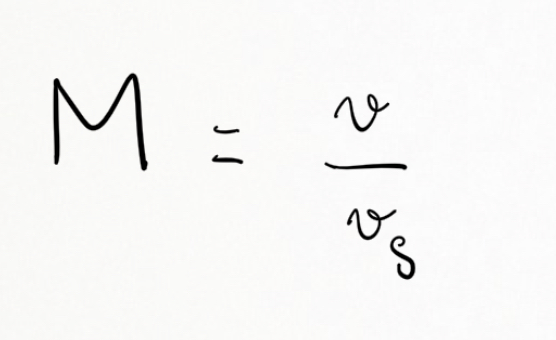MCAT Content / Sound / Shock Waves

### Shock Waves

Topic: Sound

Shock waves are a constructive interference of sound created by an object moving faster than sound.

If the source exceeds the speed of sound, no sound is received by the observer until the source has passed. Consequently, the sounds from the approaching source are mixed with those from it when receding. This mixing appears messy, but something interesting happens—a shock wave is created.Constructive interference along the lines shown (a cone in three dimensions) from similar sound waves arriving there simultaneously. This superposition forms a disturbance called a shock wave, constructive interference of sound created by an object moving faster than sound. Inside the cone, the interference is mostly destructive, so the sound intensity there is much less than on the shock wave.

You may have heard the term Mach number in relation to the speed of spacecrafts or jets before. This is a ratio of an object’s speed in relation to the speed of sound. The Mach number is the speed of the source divided by the speed of sound:where M is the Mach number, V is the velocity of the object, and V is the speed of sound in the medium. If something is traveling at the speed of sound, the Mach number would be equal to 1 and can be denoted as Mach 1.

Key Points

• When an object moves at a speed equal to the speed of sound, or faster, shock waves are formed by a sudden rise and fall of air pressure,

• Shock waves are visible because the sudden decrease in air pressure in them caused water molecules in the air to condense

Key Terms

Mach number: The ratio of an object’s speed in relation to the speed of sound.

Supersonic: Faster than the speed of sound (approximately 343.2 m/s)

Constructive interference: The interference of two or more waves of equal frequency and phase, resulting in their mutual reinforcement and producing a single amplitude equal to the sum of the amplitudes of the individual waves.

Shock wave: A sharp change of pressure in a narrow region traveling through a medium, especially air, caused by explosion or by a body moving faster than sound.

Billing Information